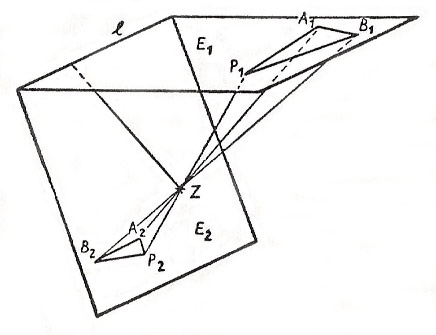A# perspectiveFigure 2.

Two figures or solid bodies that arise from one another by a perspective transformation are said to be in perspective with respect to one another. The perspective image of a circle can, for example, be a parabola, and the parabola and the circle are then in perspective with respect to one another.

## Perspective transformation

### Plane perspective transformation

In a perspective transformation in a plane, the points of two different straight lines are associated with one another in the following way:

If l and m are given straight lines and Z is a point of the plane which lies neither on l or m, then a point L of l is associated with the point of intersection, M, of ZL with M (M is the image-point of L). Z is called the perspective center or the center of perspectivity (see Figure 1). Thus in a perspective, the image-points on m arise out of the points of l by central projection.Figure 1.

Such a perspective of the points of two straight lines possesses a fixed point (a point whose image coincides with the point itself), namely the point of intersection P of the two lines.

## Example of perspective transformation in space

If E1 and E2 are two different planes in space, and Z is a point outside both E1 and E2, a perspective can be defined in the following way.

If P1 is a point of E1 the point of intersection P2 of the line ZP1 with the plane E2 is associated with P1 as its image-point. The points of the line of intersection l of E1 and E2 are fixed points of this transformation (see Figure 2).